Next: Graph Theory Based Algorithms Up: Geometric Based Algorithms Previous: Coordinate bisection

### Inertia bisection

Partitioning using coordinate bisection is susceptible to the orientation of the mesh, a simple rotation of the mesh would result in different partition. Inertia bisection  remedies this by using a procedure that is invariant to rotation. It finds the principal axis of the communication graph. Here the coordinates of the vertices of the communication graph are defined as explained in Section 3.1.1. If one assumes that each vertex has a unit mass associated with it, then the principal axis is the axis that minimizes the angular momentum when the graph rotates around it.

Consider a graph of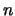vertices associated with a 3D mesh. Let the principal axis bewitha point in space,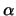real numbers and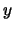a unit vector. Then any vertex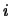with coordinateshas an angular momentum equal to the square of its distance to the axis, or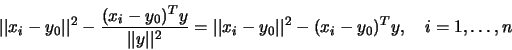The principal axis minimizes the sum of these momentums: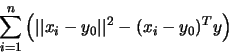subject to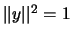. It is possible to prove, using the necessary condition of constrained optimization, that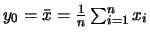and thatis the eigenvector that corresponds to the largest eigenvalue of the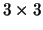matrix(1)

Once this eigenvector has been found, every vertex can be sorted based on its projection onto the principal axis, that is, the value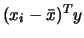, or simply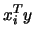.

The main computational cost of the inertia algorithm is the formation of the inertia matrix (1), which requires of the order of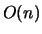operations. The algorithm is easy to parallelize [18,74].Next: Graph Theory Based Algorithms Up: Geometric Based Algorithms Previous: Coordinate bisection

2000-03-21